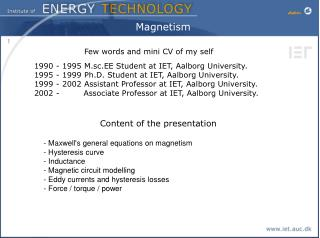Download PresentationMagnetismMagnetism - PowerPoint PPT Presentation

Download PresentationMagnetism
An Image/Link below is provided (as is) to download presentation

Download Policy: Content on the Website is provided to you AS IS for your information and personal use and may not be sold / licensed / shared on other websites without getting consent from its author. While downloading, if for some reason you are not able to download a presentation, the publisher may have deleted the file from their server.

- - - - - - - - - - - - - - - - - - - - - - - - - - - E N D - - - - - - - - - - - - - - - - - - - - - - - - - - -
Presentation Transcript

1. Magnetism Few words and mini CV of my self 1990 - 1995 M.sc.EE Student at IET, Aalborg University. 1995 - 1999 Ph.D. Student at IET, Aalborg University. 1999 - 2002 Assistant Professor at IET, Aalborg University. 2002 - Associate Professor at IET, Aalborg University. Content of the presentation - Maxwell's general equations on magnetism- Hysteresis curve- Inductance- Magnetic circuit modelling - Eddy currents and hysteresis losses- Force / torque / power

2. Maxwell James Clerk Maxwell 1831 - 1879 Unified theory of the connection between electricity and magnetism

3. Maxwells equations in free space Not easy to understand in this form. Practical engineers tend to forget the definition of divergence, curl, surface and line integral

4. Ørsted H.C Ørsted 1777-1851 1820 Ørsteds discovery was a deflection of a Compass needle when it is close to a current carrying conductor. But he was not able to describe the physics or mathematics behind the phenomena {f = Bxil / 90° : f=Bil}

5. Amperé André-Marie Ampére 1775 – 1836 Just one week after Ørsteds discovery Ampere showed that two current carrying wires attract or repulse each other, and was able to show that : I1 r I2 l Thus, for two parallel wires carrying a current of 1 A, and spaced apart by 1 m in vacuum, the force on each wire per unit length is exactly 2 × 10-7 N/m. It was first in 1826 he published the circuit law in Maxwell’s equation

6. Faraday The Induction law 1821 Michael Faraday 1791-1867 {e = Bxlv / 90° : e =Blv} Induction by movement ”generator” Induction by alternating current ”transformer”

7. Gauss Carl Friedrich Gauss 1777 – 1855 Basically the magnetic induction can’t escape. The equation says that we don’t have a monopole. There will always be a north and a south pole

8. Relations in Maxwell’s equations Simplified analogies and the relations in Maxwell’s equations

9. Permeability Return μ =μr μ0 Where μr typically is 1000-100000 in magnetic cores

10. Analogies

12. Some of the first electrical machines Alternator by Pixii 1832 Not useful because it makes AC Improved to a DC machine where Ampere proposed Pixii to use a mechanical commutator Note it is made with electromagnets (Henry 1828)

13. Letz’s law Letz’s law (consequence of Maxwell’s equation)

14. Amperé’s law Ampere’s law (again)

15. Inductor example Example : Inductor

16. Inductor example

17. Inductor example No saturation and hysteresis

18. Magnetic circuit model Magnetic circuit model Ohm’s law in magnetic circuits

19. Magnetic circuit model What about Kirchoff’s current law (KCL) Gauss law

20. Magnetic circuit model What about Kirchoff’s voltage law (KVL) Ampere law is similar to Kirchoff’s voltage law

21. Magnetic circuit model (Steel and air-gap) Magnetic circuit model (Steel and air-gap)

22. Magnetic circuit model (Steel and air-gap)

23. Core losses Hysteresis losses

24. Hysteresis losses Hysteresis losses

25. Hysteresis losses Hysteresis curve at various H-fields

26. Hysteresis losses

27. Eddy current losses Eddy current losses

28. Eddy current losses How do we reduce Eddy current losses SOLID LAMINATED

29. Eddy current losses Eddy current losses

30. Eddy current losses in windings Eddy current losses in windings Can be a problem with thick wires - Low voltage machines - High speed machines

31. Force, torque and power Universal modeling of terminal characteristic of electro-magnetic devices based on energy balance

32. Force, torque and power

33. Force, torque and power

34. Force, torque and power

35. Force, torque and power

36. Force, torque and power

37. Force, torque and power

38. Force, torque and power

39. Force, torque and power

40. Force, torque and power

41. Simplified machines

42. Simplified machines

43. Electromagnetic gearing Lgu larger than Lg≈ ∞ Δθ=90

44. Electromagnetic gearing Same winding but now enclosing two poles : LA is the same (if end winding is neglected the Resistance is also the same) Δθ=45 Torque doubling

45. Normal/radial forces Normal forces Ex. Changing the air gap from 0.3 mm to 0.15 mm Gives a doubling of inductance The Δx is very small i.e a large Force In electrical machines the normal forces versus the tangential force (torque) is typically a factor 10.